# 虎星 (暗影幻象)

Tigerstar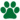独行猫 (Q648)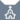守护者 (Q652)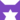星族 (Q634)[濒死]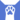反抗者 (Q4414)

（继任自花楸掌 (Q2692)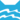河族 管理者
（继任自枭鼻 (Q2332)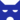影族 学徒影族 武士影族 副族长
（继任自乌霜 (Q3211)守护者 守护者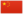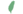小虎 Tigerkit  小虎
 虎爪 Tigerpaw  虎掌
 虎心 Tigerheart  虎心
 虎星 Tigerstar  虎星

Q2687：影族公猫，自《暗河汹涌》登场的角色

## 细节

### 外貌

• 他是一只身形庞大、:95肩膀宽阔、:17皮毛光滑:251厚实、:76:105琥珀色眼睛、:196暗棕色:猫物表虎纹:128的虎斑:猫物表公猫。:128他有一条长尾巴，:96:封面耳撕裂。:9章
• 他的外貌和舅舅黑莓星极为相似。:177
• 而黑莓星又被描述为其父虎星:6章和弟弟鹰霜:10章的分身，亦即为虎星看上去也与外祖父虎星和小舅舅鹰霜极为相似。

## 登场书目

• 暗河汹涌 (Q153)[对话提及][首次登场🐱]
• 驱逐之战 (Q154)[配角]
• 天蚀遮月 (Q155)[配角]
• 暗夜长影 (Q156)[配角]
• 拂晓之光 (Q157)[对话提及]
• 第四学徒 (Q158)[配角]
• 战声渐近 (Q159)[配角]
• 暗夜密语 (Q160)[配角]
• 月光印记 (Q161)[配角]
• 武士归来 (Q162)[配角]
• 群星之战 (Q163)[配角]
• 学徒探索 (Q170)[配角]
• 雷影交加 (Q171)[配角]
• 天空破碎 (Q172)[配角]
• 极夜无光 (Q173)[配角]
• 烈焰焚河 (Q174)[配角][死亡时刻✝]
• 风暴来袭 (Q175)[配角]
• 迷失群星 (Q3563)[配角]
• 静默冰融 (Q3579)[配角]
• 叠影重障 (Q3624)[配角]
• 暗由心生 (Q4183)[配角]
• 无星之地 (Q4385)[配角]
• 迷雾明光 (Q4621)[配角]
• 河翻浪涌 (Q4643)[配角]
• 天穹将倾 (Q4799)[配角]
• 黑莓星的风暴 (Q182)[配角]
• 虎心的阴影 (Q185)[主人公][主要角色][视角人物][死亡时刻✝]
• 鸦羽的拷问 (Q186)[猫物闲角]
• 松鼠飞的希冀 (Q3561)[配角]
• 灰条的誓言 (Q4057)[猫物闲角]
• 一星的告解 (Q4826)[配角]
• 冬青叶的故事 (Q187)[猫物闲角]
• 雾星的征兆 (Q188)[猫物闲角]
• 鸽翅的沉默 (Q192)[配角]
• 褐皮的族群 (Q3566)[配角]
• 黛西的至亲 (Q3629)[猫物闲角]
• 斑毛的反抗 (Q4337)[配角]
• 族群的战争 (Q202)[配角]
• 终极指南 (Q204)[对话提及]

## 脚注

1. 他强调自己不是也不会成为河族的族长，故列为管理者一职。

## 参考文献

这篇文章基于CC BY-SA 3.0许可使用了猫武士维基（英语）Tigerstar (AVoS)一文中的部分内容。
1. 《暗河汹涌》第一章 (Q1098)
2. 河翻浪涌 (Q4643)
3. 暗夜长影
4. 暗夜密语
5. 战声渐近
6. 群星之战
7. 日落和平
8. 驱逐之战
9. 影瘴迷心
10. 极夜无光
11. 力挽狂澜
12. 重现家园
13. 风起云涌
14. Su Susann (2016-09-30). 站内截图. Facebook . 原页面 归档于 2017-10-31. “As I said, Adderfang is a descandant of Spottedpelt and Pinestar is, trough the blood of his father, related to Gorseclaw.”
15. 黄牙的秘密
16. 艾琳·亨特访谈7
17. 虎心的阴影
18. 蛾飞的幻象
19. 烈焰焚河
20. 风暴来袭
21. 天穹将倾
1. 在同一份由维琪授意、Su所公布的黑森林名单中，官网家谱已将雪暴翅、亮花和沼云标注为星族成员，短尾标注为黑森林成员；鹿脚、百合须和缠果的死后去处则暂时没有更进一步的资讯。